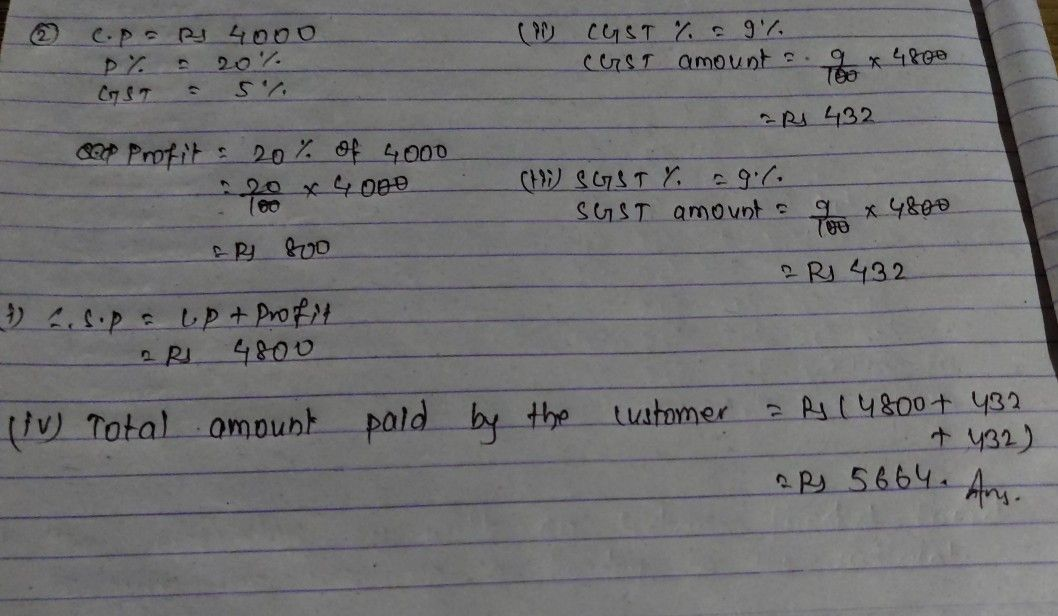Symbol
Problem$-$ A shopkeeper buy goods worth 7 $-7$ and sells these at $=5$ profit of $7$ $-$ $2$ $5$ consuer in the same state. If $-=$ is charged at $5$ find: e the selling price (excluding tax) of the goods.t00 $7$ $-$ $\square 352$ paid by the consumer. $-$ $2$ paid by the consumer. (i) the total amount paid by the consumer $4==$ $=$
10th-13th grade
Algebra
Search count: 105
SolutionQanda teacher - pawan